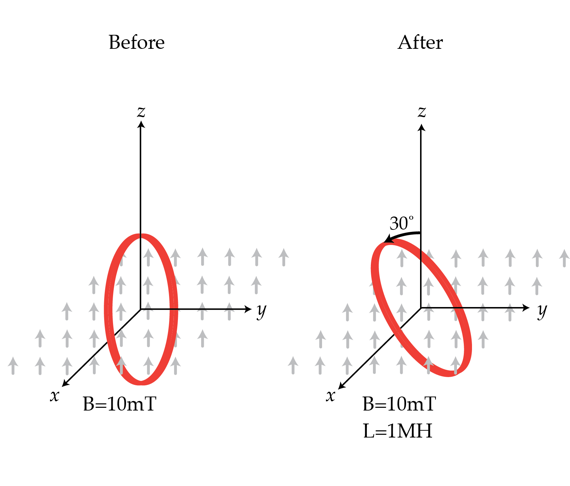# Rotating a superconducting loop

A superconducting (zero resistivity) loop of area $A=10^{-2} m^2$ is placed in a constant magnetic field of induction $B=10 mT$ so that its plane is parallel to the magnetic field. The ring is now rotated so that the magnetic field forms an angle of $30^{\circ}$ with its plane. What will be the current in Amps in the loop after it is rotated if its inductance is $L=1 \mu H$?×

Problem Loading...

Note Loading...

Set Loading...